Скачать презентацию Algebraic Expressions What is a Variable

ccb705098a27554ea2bf99e7e8bc21c4.ppt

• Количество слайдов: 10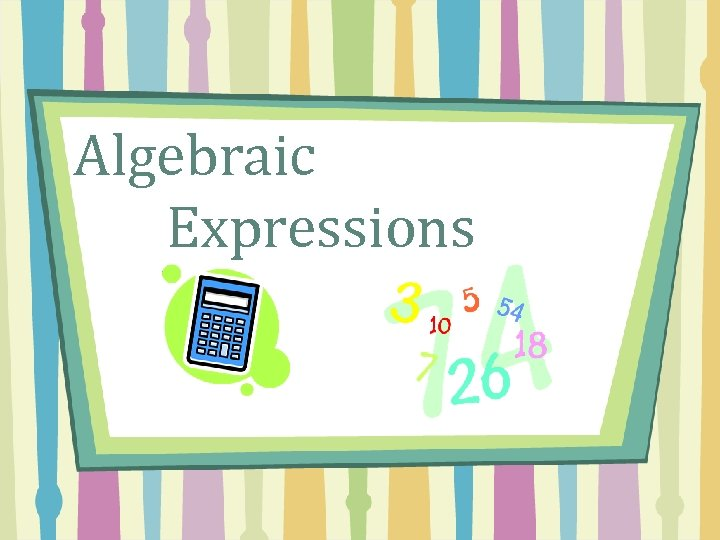Algebraic Expressions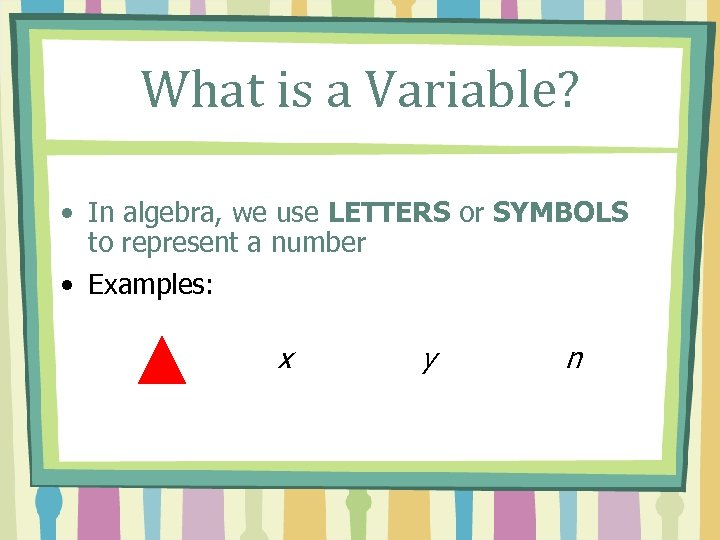What is a Variable? • In algebra, we use LETTERS or SYMBOLS to represent a number • Examples: x y n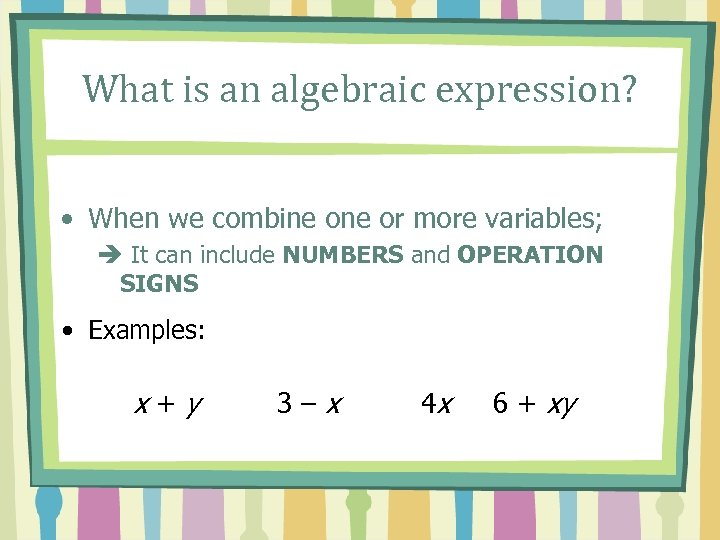What is an algebraic expression? • When we combine or more variables; è It can include NUMBERS and OPERATION SIGNS • Examples: x+y 3–x 4 x 6 + xy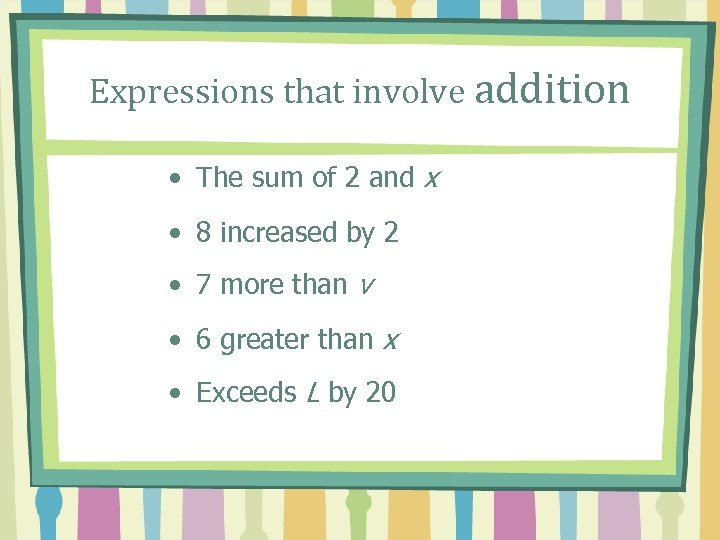Expressions that involve addition • The sum of 2 and x • 8 increased by 2 • 7 more than v • 6 greater than x • Exceeds L by 20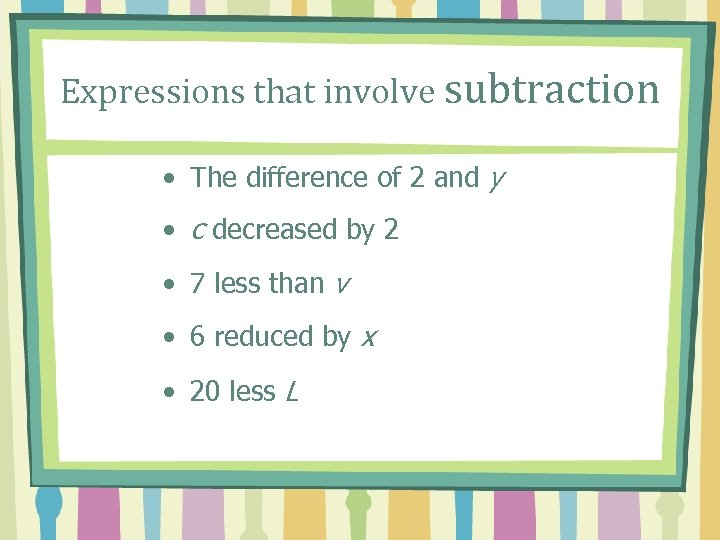Expressions that involve subtraction • The difference of 2 and y • c decreased by 2 • 7 less than v • 6 reduced by x • 20 less L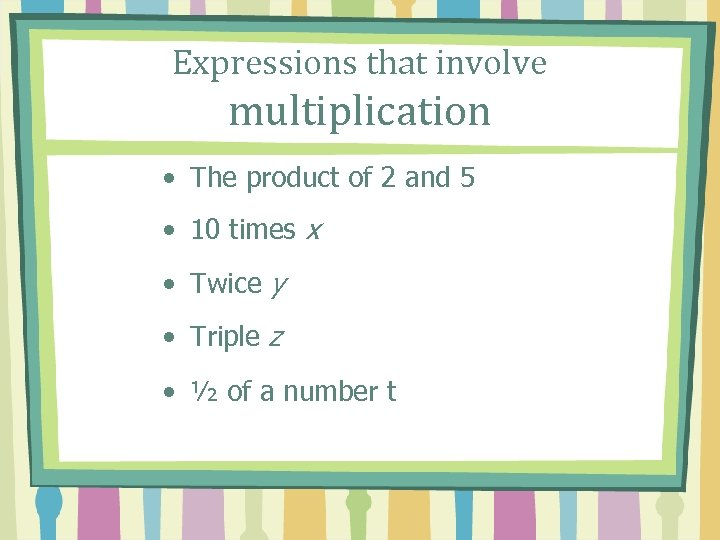Expressions that involve multiplication • The product of 2 and 5 • 10 times x • Twice y • Triple z • ½ of a number t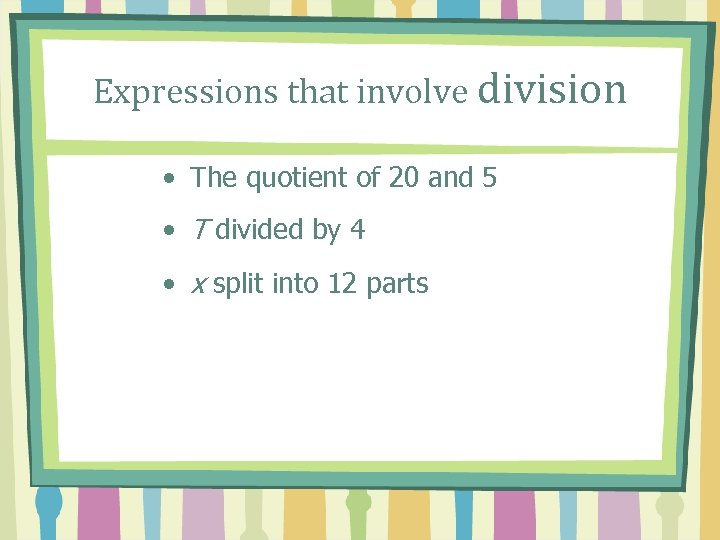Expressions that involve division • The quotient of 20 and 5 • T divided by 4 • x split into 12 parts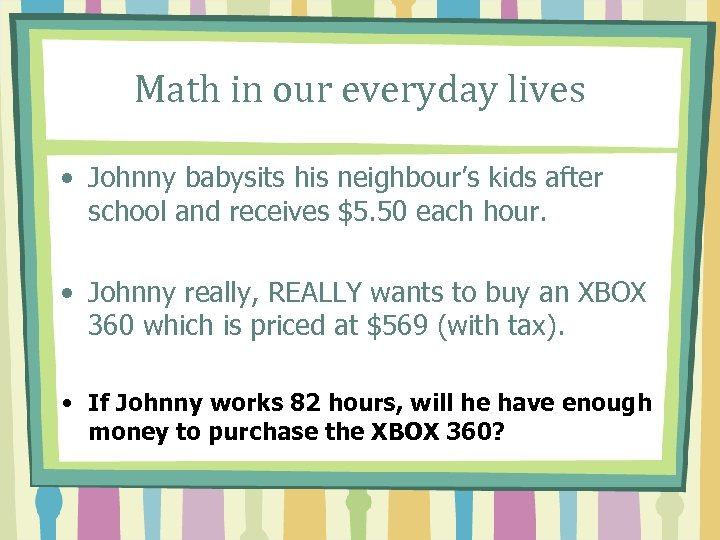Math in our everyday lives • Johnny babysits his neighbour’s kids after school and receives \$5. 50 each hour. • Johnny really, REALLY wants to buy an XBOX 360 which is priced at \$569 (with tax). • If Johnny works 82 hours, will he have enough money to purchase the XBOX 360?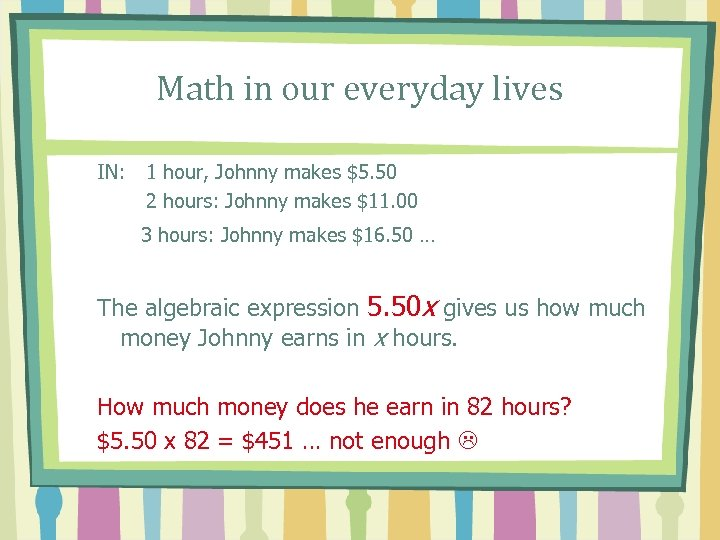Math in our everyday lives IN: 1 hour, Johnny makes \$5. 50 2 hours: Johnny makes \$11. 00 3 hours: Johnny makes \$16. 50 … The algebraic expression 5. 50 x gives us how much money Johnny earns in x hours. How much money does he earn in 82 hours? \$5. 50 x 82 = \$451 … not enough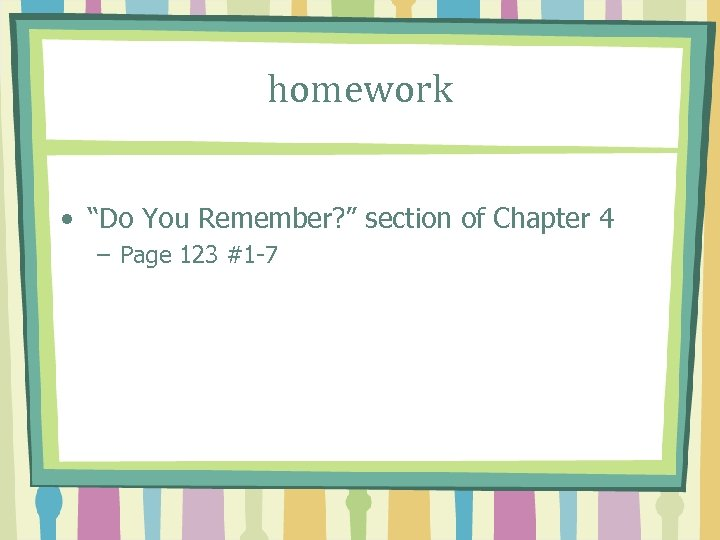homework • “Do You Remember? ” section of Chapter 4 – Page 123 #1 -7